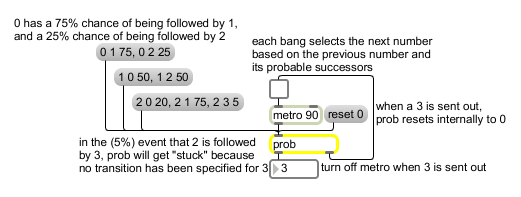prob

Make a weighted random series of numbers

Description

prob accepts lists of three numbers in its inlet. The third number represents the weight of the probability of going from states represented by the first two numbers. For example, 1 2 4 would mean that there is a weight of 4 in going from state 1 to state 2. When prob receives a bang, it makes a random jump from its current state to another state based on its current weighting of transitions. If a transition can be made, the new state is sent out the left outlet. If not, a bang is sent out the right outlet. For any particular state, the weights of all possible transition states are summed. Thus if a state could jump to three states that had weights of 3 4 and 1, the first one (3) would occur 37.5% of the time, the second 50% of the time, and the third 12.5%. Note that any state can make a transition to itself with a list of the form (state state weight).

None.

Messages

 bang Makes a weighted random choice of a number to be sent out, based on the immediately previous output and on the specified likelihoods of subsequent numbers. No output will be produced if no input has been received or if the contents of the prob object have been cleared using the clear message. int input [int] Sets (but does not send out) out the current number value. The subsequent output, in response to a bang message, will be determined by the stored matrix of probable transitions from that number. list probability-matrix-of-transitions [list] The numbers make an entry in a probability matrix of transitions from one number to another (known as a first-order Markov chain). The list should consist of three numbers: a current value, a next value, and a probability that current will be followed by next. The first two numbers in the list identify a possible succession of output values: a possibility that the first number will be followed by the second. The third number sets the relative likelihood that the sequence of numbers will occur. Once the first number has been sent out, the next output is determined by the relative likelihood(s) assigned to each possible subsequent number. clear Erases the contents of the prob object. embed save-with-patch-flag (0 or non-zero) [int] The word embed, followed by a non-zero number, causes the contents of prob to be saved as part of the patch that contains it. The message embed 0 causes prob to forget its contents when the patch is closed. dump Prints out a complete list of the stored transition probabilities (Markov chain) in the Max window. reset restart-value [int] The word reset, followed by a number, tells prob what number to revert to in the event that it gets "stuck" on a number that has no possible next number.

Output

bang: Out right outlet: If the current number (the last number chosen) has no possible transitions listed in the transition probability matrix, bang is sent out (and nothing is sent out the left outlet) in response to a bang in the inlet.
int: Out left outlet: When bang is received in the inlet, prob sends out a number, which it chooses based on its knowledge of the last number chosen and the relative likelihood assigned to each possible subsequent number.

ExamplesLikelihood of a certain output depends on the previous output